#There are 366 different Starters of The Day, many to choose from. You will find below some starters on the topic of Statistics. A lesson starter does not have to be on the same topic as the main part of the lesson or the topic of the previous lesson. It is often very useful to revise or explore other concepts by using a starter based on a totally different area of Mathematics.

Main Page

### Statistics Starters: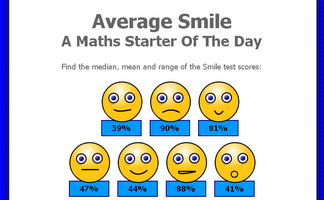Calculate the mean, median and range of the given scores.An exercise in estimating what the sectors of a pie chart represent.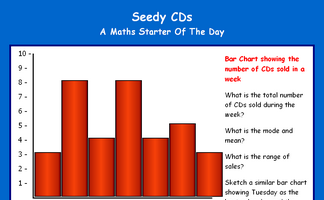Interpret data given in the form of a bar chart then create a new bar chart showing the given statistics.Eight mathematical questions about the current weather and times in four cities around the world.

## Exercises#### A Show Of Hands

Produce a number of graphs and charts from a quick show of hands.#### Averages

Test your understanding of averages with this self marking quiz about mean, median and range.#### Box Plots

An exercise on reading and drawing box-and-whisker diagrams which represent statistical data.This is a game for two players. You should know how to find the mean, median and range of a set of numbers.#### Code Cracker

Crack the code by replacing the encrypted letters in the given text. There are lots of hints provided about code breaking techniques.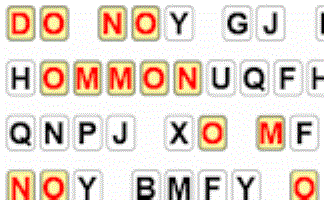#### Code Cracking Presentation

A slide presentation showing techniques for cracking simple codes and ciphers.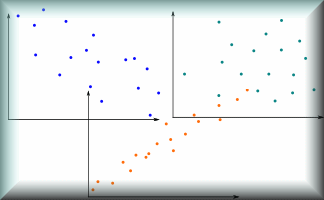#### Correlation

Arrange the given statements in groups to show the type of correlation they have.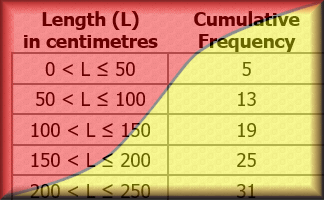#### Cumulative Frequency

Online exercises on cumulative frequency for discrete and grouped data with exam-style questions.#### Estimating Correlation

Practise the skill of estimating the correlation of data on a scatter graph in this self marking exercise.#### First Impressions

This activity will collect data about your first impressions of some optical illusions. You can then analyse the data to come to your own conclusions.#### Histograms

Practise drawing and reading information from histograms displaying grouped data#### Human Scatter Graphs

Pupils move to positions in the room according to their data relative to the walls as axes.#### Normal Distribution Calculator

A customised online calculator for quickly finding areas under the normal distribution curve.#### Pie Chart Creator

A quick and convenient tool for rapidly creating simple pie charts.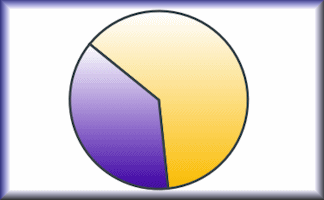#### Pie Charts

Develop the skills to construct and interpret pie charts in this self-marking set of exercises.#### Pin Drop

Estimate the probability of a drawing pin landing point up from experimental data.A simulation of a Quincunx (Galton Board) which can be used to create the bell shaped curve of the normal distribution.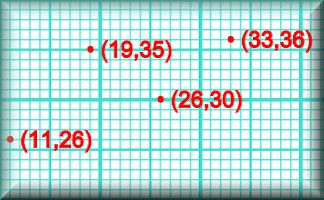#### Plotting Scatter Graphs

Plot scatter graphs from data representing a number of different everyday situations.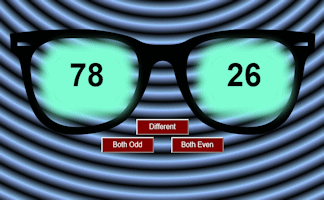#### Reaction Time

When the numbers appear hit the correct button depending on whether the numbers are even or odd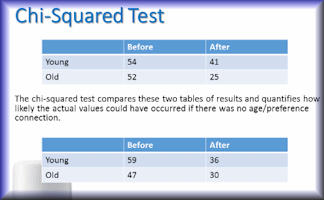#### Significance

A slide presentation showing how to use the chi-squared test to measure significance.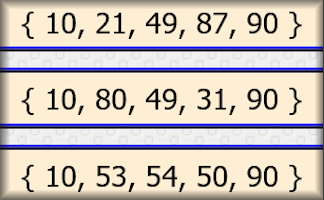#### Standard Deviation

Find how 'spread out' data is by estimating and calculating this measure of dispersion.#### t-Test Revision

A slide presentation designed to revise the key aspects of Student's t-Test.

### Search

The activity you are looking for may have been classified in a different way from the way you were expecting. You can search the whole of Transum Maths by using the box below.

Have today's Starter of the Day as your default homepage. Copy the URL below then select
Tools > Internet Options (Internet Explorer) then paste the URL into the homepage field.

Set as your homepage (if you are using Internet Explorer)

Do you have any comments? It is always useful to receive feedback and helps make this free resource even more useful for those learning Mathematics anywhere in the world. Click here to enter your comments.For All: